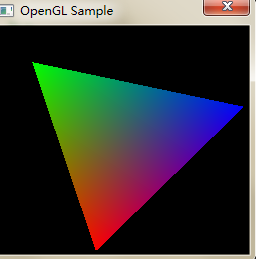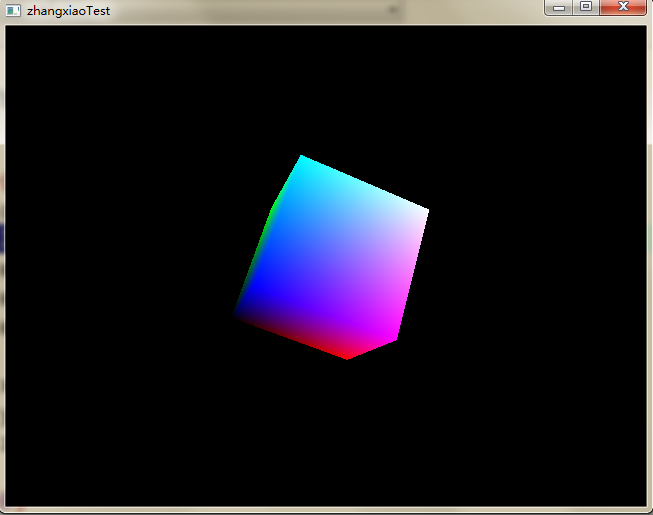# Implementation of OpenGL rotating cube

` `

Topic request
OpenGL is used to implement a rotating cube.

Process steps
It is mainly divided into two parts: setting up the environment and completing the code writing.
Set up the environment
`TDM GCC 4.9.2`code>TDM GCC 4.9.2 `TDM GCC 4.9.2` `TDM GCC 4.9.2` `TDM GCC 4.9.2`

``` File - & gt; New - & gt; Project - & gt; Multimedia -> OpenGL directly compile and run the Demo, which will show a rotating triangle as shown in the image below:> > > Will be relevant. DLL file copy to the C: \ Windows \ system32 directory the related. Lib file copy to D: \ \ Program Files \ Dev - Cpp \ MinGW64 \ x86_64 - w64 - mingw32 \ lib32 directory the related. H file copy to D: \ \ Program Files \ Dev - Cpp \ MinGW64 \ include \ GL directory the last link is added in the compiler options, Set the appropriate compilation options under Linux and in the Makefile. ```

```Draw cube void cube()/ code>void cube() glBegin()``` and > glEnd()e> > `glColor3f()>>glVertex3f()e> /code> ` glVertex3f() glVertex3f() glVertex3f()

``````void cube()
{
glColor3f(1.0,1.0,0.0);
glVertex3f( 1.0, 1.0,-1.0);
glColor3f(0.0,1.0,0.0);
glVertex3f(-1.0, 1.0,-1.0);
glColor3f(0.0,1.0,1.0);
glVertex3f(-1.0, 1.0, 1.0);
glColor3f(1.0,1.0,1.0);
glVertex3f( 1.0, 1.0, 1.0);

glColor3f(1.0,0.0,1.0);
glVertex3f( 1.0,-1.0, 1.0);
glColor3f(0.0,0.0,1.0);
glVertex3f(-1.0,-1.0, 1.0);
glColor3f(0.0,0.0,0.0);
glVertex3f(-1.0,-1.0,-1.0);
glColor3f(1.0,0.0,0.0);
glVertex3f( 1.0,-1.0,-1.0);

glColor3f(1.0,1.0,1.0);
glVertex3f( 1.0, 1.0, 1.0);
glColor3f(0.0,1.0,1.0);
glVertex3f(-1.0, 1.0, 1.0);
glColor3f(0.0,0.0,1.0);
glVertex3f(-1.0,-1.0, 1.0);
glColor3f(1.0,0.0,1.0);
glVertex3f( 1.0,-1.0, 1.0);

glColor3f(1.0,0.0,0.0);
glVertex3f( 1.0,-1.0,-1.0);
glColor3f(0.0,0.0,0.0);
glVertex3f(-1.0,-1.0,-1.0);
glColor3f(0.0,1.0,0.0);
glVertex3f(-1.0, 1.0,-1.0);
glColor3f(1.0,1.0,0.0);
glVertex3f( 1.0, 1.0,-1.0);

glColor3f(0.0,1.0,1.0);
glVertex3f(-1.0, 1.0, 1.0);
glColor3f(0.0,1.0,0.0);
glVertex3f(-1.0, 1.0,-1.0);
glColor3f(0.0,0.0,0.0);
glVertex3f(-1.0,-1.0,-1.0);
glColor3f(0.0,0.0,1.0);
glVertex3f(-1.0,-1.0, 1.0);

glColor3f(1.0,1.0,0.0);
glVertex3f( 1.0, 1.0,-1.0);
glColor3f(1.0,1.0,1.0);
glVertex3f( 1.0, 1.0, 1.0);
glColor3f(1.0,0.0,1.0);
glVertex3f( 1.0,-1.0, 1.0);
glColor3f(1.0,0.0,0.0);
glVertex3f( 1.0,-1.0,-1.0);
glEnd();
}``````

The cube rotates about its axis
In OpenGL, `glRotatef()`nction is used to achieve rotation at a specific Angle. However, to achieve rotation, it is necessary to keep changing the parameters of rotation Angle. Here, we control the rotation of the cube around three axes by setting three static variables and allowing them to increase automatically, and control the rotation speed by changing the increment.

``````static float xrot = 0.0;
static float yrot = 0.0;
static float zrot = 0.0;
glRotatef(xrot, 1, 0, 0);
glRotatef(yrot, 0, 1, 0);
glRotatef(zrot, 0, 0, 1);
cube();
xrot = xrot + 0.01;
yrot = yrot + 0.01;
zrot = zrot + 0.01;``````

The cube moves along an axis
` glTranslatRef ()`nction Specifies the position of the cube in the 3D coordinate system in OpenGL. Here let’s take the Z axis as an example and let the cube move back and forth, where H is used to control the speed of motion:

``````static float z=-5;
static float h=-0.001;
glTranslatef(0, 0, z);
z=z+h;
if(z<-10)
h=-h;
if(z>-5)
h=-h;``````

This completes the simple rotation and axis movement of the cube.

The problem

mainly compiler options. At the same time, references the glut in lib and glut32 lib, and ` in the compiler options - lglut - lglut32 front, the linker when first looking for OPENGL. DLL without looking for opengl32. DLL, at the moment we entered the DEV in c + + project configuration in the face of the changes:`

``` Project - & gt; Project Properties ->; Parameters - & gt; Link - lopengl32 - lglut32// in front - lglut - LGLU - lglu32 It compiles correctly and runs as expected:tips It took a week to finally complete the computer graphics such a simple big job - to achieve the rotation of the cube. in the code level, is very simple, because of the content is not much involved, and provided a good OpenGL function encapsulation, the Internet has a lot of similar sample. The most troublesome part is the environment construction, because OpenGL is an open source project, there is no very authoritative manual guide, so the online case is also good and bad, learning efficiency is very low. in the beginning, for example, to find a project, use the Glaux , finally you can compile all that trouble found that can't run, then online and others said the Glaux basic deprecated , and Windows 7 didn't seem to support, had to start from scratch. ```
``` Read More: An example of drawing rotating cube with OpenGL Drawing cube with OpenGL Drawing a cube with OpenGL OpenGL cube texture map Stm32cube ide installation – NSIS error problem OpenGL learning — problems encountered in compiling OpenGL program for the first time ERROR command failed: npm install –loglevel error vue-cli-plugin-cube-ui -D Implementation of screen cleaning in Python idle Cocos creator 2.4.5 2D RPG (Infinite scrolling background implementation) Python implementation of bilibilibili (B station) video download Several implementation methods of Python timing task Implementation of multithread sequential alternate execution by using lock in Java Implementation of Python switch / case statements 43. Implementation of the shortest code of multiply strings | Java Design and implementation of music website based on Java Python switch / case statement implementation method OpenGL basic graphics programming – OpenGL and 3D graphics world Square root of X (implementation of binary search) Implementation of tupledesc and tuple in mit6.830 lab1 / exercise 1 Implementation of HTTPS file server based on nginx in win2012 (adsbygoogle = window.adsbygoogle || []).push({}); ```
``` This entry was posted in How to Fix and tagged C/C++, OpenGL on 2021-02-13 by Robins. ```
``` Post navigation ← Drawing a cube with OpenGL OpenGL cube texture map → ```